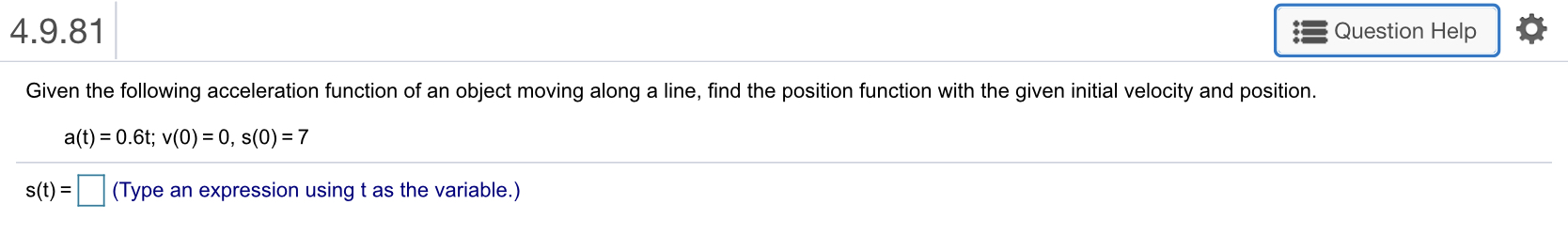# 4.9.81Question HelpGiven the following acceleration function of an object moving along a line, find the position function with the given initial velocity and position.a(t) = 0.6t; v(0) = 0, s(0)-7s(t)-□ (Type an expression using t as the variable.)

Question
219 viewshelp_outlineImage Transcriptionclose4.9.81 Question Help Given the following acceleration function of an object moving along a line, find the position function with the given initial velocity and position. a(t) = 0.6t; v(0) = 0, s(0)-7 s(t)-□ (Type an expression using t as the variable.) fullscreen
check_circle

Step 1

We have to find position function from given acceleration function and given intial condition.

Functiona and initial condition is given below:

Step 2

We will use following rules to find integration.

Rules are given below:

Step 3

As we know that acceleration function is derivative of velocity function ,so with the help of integarion we can easily find velocity...

### Want to see the full answer?

See Solution

#### Want to see this answer and more?

Solutions are written by subject experts who are available 24/7. Questions are typically answered within 1 hour.*

See Solution
*Response times may vary by subject and question.
Tagged in

### Calculus